# Absolute Value of a Number

## Introduction

In this article we are going to see the absolute value function of a number, what are its properties, some graphics and as an extra we are going to see how to calculate the absolute value of an expression in the Unity graphic engine, in the field of programming and game development.

## Definition of the Absolute Value of a Number

The absolute value of a number is the distance between that number and the 0 of the measurement system.

This results in two possible outcomes depending on the number in question.

If the number is positive, the result of taking the absolute value is the same number. If on the other hand the number is negative, when taking the absolute value the result is the same number but in positive value.

Formally: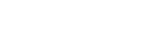Because of this definition we can affirm that the absolute value of a number is always a positive number.

## Graph of the Absolute Value of X

Below are some graphs of the absolute value function to understand how it behaves.

Observe in each graph the ranges of the variables in the axes to see how the graph is affected by the displacements in X in Y and by the coefficients that multiply the expression.

(1)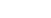(2)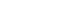(3)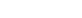(4)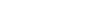## Distances

When we are working with different points in a coordinate system, we are often interested in working with the distances between them, which are positive values.

The absolute value allows working with these distances in mathematical expressions.

## How to calculate the Absolute Value in Unity

To calculate the absolute value of an expression in Unity, we must make use of the static method “Abs” of the class “Mathf”.

Let “num” be a numerical variable defined in the program in question, to take its absolute value we do:

Mathf.Abs(num);

The result of the execution of this method can be stored in another variable or in the same one, in the following way:

num = Mathf.Abs(num);